login  home  contents  what's new  discussion  bug reports help  links  subscribe  changes  refresh  edit

Anonymous shared the following library of statistical functions with us. Many thanks! (He added, that they are not checked...)

fricas
)abbrev package STAT StatPackage
++ Description:
++ This package exports statistic utilities
StatPackage(S,A) : Exports == Implementation where
S: SetCategory
A: Join(Collection(S), finiteAggregate)
Exports == with
if A has FiniteLinearAggregate(S) and S has OrderedSet then
median: A -> S
++ median(a) median of a collection (ordering from OrderedSet)
if S has Field then
mean: A -> S
++ mean(a) arithmetic mean of a collection
hmean: A -> S
++ hmean(a) harmonic mean of a collection
moment: (A,Integer) -> S
++ moment(a,i) i nth moment of a collection
variance: A -> S
++ variance(a) variance of a collection
center: A -> A
++ center(a) center collection
if A has shallowlyMutable then
center!: A -> A
++ center!(a) center collection
gmean: A -> S
++ gmean(a) geometric mean of a collection
stdev: A -> S
++ stdev(a) root mean square of a collection
stdize: A -> A
++ stdize(a) standardize a collection
if A has shallowlyMutable then
stdize!: A -> A
++ stdize!(a) standardize a collection
if A has FiniteLinearAggregate(S) and S has OrderedSet then
median(m)==
n := sort(m)
i:= divide(#m,2)
if (zero? i.remainder) then
j:= elt(n,i.quotient+minIndex(m)-1)
else
j:= elt(n,i.quotient+minIndex(m))
j
if S has Field then
if A has OneDimensionalArrayAggregate(S) then
sums: A ->List S
sums(m)==
j:=0::S
j2:=0::S
for i in minIndex(m)..maxIndex(m) repeat
h:= elt(m,i)
j := j + h
j2 := j2 + h*h
[j,j2]
variance(m)==
i := center(m)
s := sums(i)
k := (s.2 - s.1/#m::S)/(#m::S - 1::S)
k
stdize(m)==
i := mean(m)
j := map(xx +-> xx-i, m)
s := sums(j)
k := sqrt((s.2 - s.1/#m::S)/(#m::S - 1::S))
map(xx +-> (xx-i)/k,m)
if A has shallowlyMutable then
stdize!(m)==
i := mean(m)
j := map(xx +-> xx - i, m)
s := sums(j)
k := sqrt((s.2 - s.1/#m::S)/(#m::S - 1::S))
map!(xx +-> (xx - i)/k, m)
else
variance(m)==
i := center(m)
j := reduce("+",i)
j2 := reduce("+", map(xx +-> xx*xx,i))
k := (j2 - j/#m::S)/(#m::S - 1::S)
k
stdize(m)==
me:= mean(m)
i := map(xx +-> xx - me, m)
j := reduce("+",i)
j2 := reduce("+", map(xx +-> xx*xx,i))
k := sqrt((j2 - j/#m::S)/(#m::S - 1::S))
map(xx +-> (xx -me)/k,m)
if A has shallowlyMutable then
stdize!(m)==
me:= mean(m)
i := map(xx +-> xx - me,m)
j := reduce("+",i)
j2 := reduce("+", map(xx +-> xx*xx, i))
k := sqrt((j2 - j/#m::S)/(#m::S - 1::S))
map!(xx +->(xx - me)/k, m)
if A has FiniteLinearAggregate(S) and S has OrderedSet then
median(m)==
n := sort(m)
min := minIndex(m)
i:= divide(#m,2)
if (zero? i.remainder) then
j:= (elt(n,i.quotient+min-1)+elt(n,i.quotient+min))/2::S
else
j:=elt(n,i.quotient+min)
j
mean(m)==
i := reduce("+", m)
(i / (#m::S))
hmean(m)==
i := map(xx +-> 1::S/xx, m)
j := reduce("+", i)
((#m::S) / j)
moment(m,n)==
n = 0 => 1
n = 1 => mean(m)
i := map(xx +-> xx^n, m)
j := reduce("+",i)
(j / (#m::S))
center(m)==
i := mean(m)
map(xx +-> xx - i, m)
if A has shallowlyMutable then
center!(m)==
i := mean(m)
map!(xx +-> xx - i, m)
m
gmean(m)==
i := reduce("*", m)
nthRoot(i,#m)
stdev(m)==
sqrt(variance(m))

fricas
)abbrev package IDSTAT IntegralDomainStatPackage
++ Description:
++ This package exports statistic utilities over IntegralDomain
IntegralDomainStatPackage(S,A) : Exports == Implementation where
S: IntegralDomain
A: Join(Collection(S), finiteAggregate)
Exports == with
mean: A -> Fraction(S)
++ mean(a) arithmetic mean of a collection
moment: (A,NonNegativeInteger) -> Fraction(S)
++ moment(m,n) nth moment of a collection
mean(m)==
i := reduce("+", m)
i / #m::S
moment(m,n)==
n = 0 => 1
n = 1 => mean(m)
i := map(xx +-> xx^n, m)
j := reduce("+",i)
j / #m::S

fricas
)abbrev package MSTAT MatrixStatPackage
++ Description:
++ This package exports statistic utilities on two dimensional arrays
++ Function are applied column by column
MatrixStatPackage(S,Row,Col,M) : Exports == Implementation where
S: SetCategory
Row : FiniteLinearAggregate S
Col : FiniteLinearAggregate S
M   : TwoDimensionalArrayCategory(S,Row,Col)
Exports == with
if Col has shallowlyMutable then
if S has OrderedSet then
median: M -> Col
++ median(a) median of a two dimensional array (ordering from OrderedSet)
if S has Field then
mean: M -> Col
++ mean(a) arithmetic mean of a two dimensional array
hmean: M -> Col
++ hmean(a) harmonic mean of a two dimensional array
variance: M -> Col
++ variance(a) variance of a two dimensional array
moment: (M,Integer) -> Col
++ moment(a,i) i nth moment of a two dimensional array
center: M -> M
++ center(a) center two dimensional array
center!: M -> M
++ center!(a) center two dimensional array
if M has MatrixCategory(S,Row,Col) then
cov:  M -> M
++ cov(a): covariance matrix
gmean: M -> Col
++ gmean(a) geometric mean of a two dimensional array
stdev: M -> Col
++ stdev(a) root mean square of a two dimensional array
stdize: M -> M
++ stdize(a) standardize two dimensional array
stdize!: M -> M
++ stdize!(a) standardize two dimensional array
if M has MatrixCategory(S,Row,Col) then
cor:  M -> M
++ cor(a): correlation matrix
import StatPackage(S,Col)
if Col has shallowlyMutable then
colReduce: (Col -> S, M) -> Col
colReduce(fx,m)==
ret : Col := new(ncols(m),NIL$Lisp) j := minColIndex(m) - 1 for i in j+1..maxColIndex(m) repeat setelt!(ret,i-j,fx(column(m,i))) ret if S has OrderedSet then median(m) == ret := colReduce(median$StatPackage(S,Col),m)
ret
if S has Field then
mean(m)==
ret := colReduce(mean$StatPackage(S,Col),m) ret hmean(m)== ret := colReduce(hmean$StatPackage(S,Col),m)
ret
variance(m)==
ret := colReduce(variance$StatPackage(S,Col),m) ret moment(m,pow)== ret : Col := new(ncols(m),NIL$Lisp)
j := minColIndex(m) - 1
for i in j+1..maxColIndex(m) repeat
setelt!(ret,i,moment(column(m,i),pow)$StatPackage(S,Col)) ret center(m)== ret : M := new(nrows(m),ncols(m),NIL$Lisp)
j := minColIndex(m) - 1
for i in j+1..maxColIndex(m) repeat
setColumn!(ret,i-j,center!(column(m,i))$StatPackage(S,Col)) ret center!(m)== j := minColIndex(m) - 1 for i in j+1..maxColIndex(m) repeat setColumn!(m,i-j,center!(column(m,i))$StatPackage(S,Col))
m
if M has MatrixCategory(S,Row,Col) then
cov(m)==
ret := center(m)
transpose(ret/(nrows(m)-1)::S) * ret
gmean(m)==
colReduce(gmean$StatPackage(S,Col),m) stdev(m)== colReduce(stdev$StatPackage(S,Col),m)
stdize(m)==
ret : M := new(nrows(m),ncols(m),NIL$Lisp) j := minColIndex(m) - 1 for i in j+1..maxColIndex(m) repeat setColumn!(ret,i-j,stdize!(column(m,i))$StatPackage(S,Col))
ret
stdize!(m)==
j := minColIndex(m) - 1
for i in j+1..maxColIndex(m) repeat
setColumn!(m,i-j,stdize!(column(m,i))$StatPackage(S,Col)) m if M has MatrixCategory(S,Row,Col) then cor(m)== ret := stdize(m) (transpose(ret/((nrows(m)-1)::S)) * ret) fricas Compiling FriCAS source code from file /var/lib/zope2.10/instance/axiom-wiki/var/LatexWiki/172717192283825040-25px.001.spad using old system compiler. STAT abbreviates package StatPackage ------------------------------------------------------------------------ initializing NRLIB STAT for StatPackage compiling into NRLIB STAT ****** Domain: A already in scope ****** Domain: A already in scope augmenting A: (FiniteLinearAggregate S) ****** Domain: S already in scope augmenting S: (OrderedSet) augmenting$: (SIGNATURE $median (S A)) compiling exported median : A -> S Time: 0.03 SEC. ****** Domain: S already in scope augmenting S: (Field) augmenting$: (SIGNATURE $mean (S A)) augmenting$: (SIGNATURE $hmean (S A)) augmenting$: (SIGNATURE $moment (S A (Integer))) augmenting$: (SIGNATURE $variance (S A)) augmenting$: (SIGNATURE $center (A A)) ****** Domain: A already in scope augmenting A: (OneDimensionalArrayAggregate S) compiling local sums : A -> List S Time: 0.02 SEC. compiling exported variance : A -> S Time: 0 SEC. ****** Domain: S already in scope augmenting S: (RadicalCategory) augmenting$: (SIGNATURE $gmean (S A)) augmenting$: (SIGNATURE $stdev (S A)) augmenting$: (SIGNATURE $stdize (A A)) compiling exported stdize : A -> A Time: 0.01 SEC. compiling exported stdize! : A -> A Time: 0 SEC. compiling exported variance : A -> S Time: 0 SEC. ****** Domain: S already in scope augmenting S: (RadicalCategory) augmenting$: (SIGNATURE $gmean (S A)) augmenting$: (SIGNATURE $stdev (S A)) augmenting$: (SIGNATURE $stdize (A A)) compiling exported stdize : A -> A Time: 0 SEC. ****** Domain: A already in scope augmenting A: (shallowlyMutable) augmenting$: (SIGNATURE $stdize! (A A)) augmenting$: (SIGNATURE $center! (A A)) compiling exported stdize! : A -> A Time: 0.02 SEC. ****** Domain: A already in scope augmenting A: (FiniteLinearAggregate S) ****** Domain: S already in scope augmenting S: (OrderedSet) augmenting$: (SIGNATURE $median (S A)) compiling exported median : A -> S Time: 0 SEC. compiling exported mean : A -> S Time: 0 SEC. compiling exported hmean : A -> S Time: 0 SEC. compiling exported moment : (A,Integer) -> S Time: 0 SEC. compiling exported center : A -> A Time: 0 SEC. ****** Domain: A already in scope augmenting A: (shallowlyMutable) augmenting$: (SIGNATURE $center! (A A)) compiling exported center! : A -> A Time: 0 SEC. ****** Domain: S already in scope augmenting S: (RadicalCategory) augmenting$: (SIGNATURE $gmean (S A)) augmenting$: (SIGNATURE $stdev (S A)) augmenting$: (SIGNATURE $stdize (A A)) compiling exported gmean : A -> S Time: 0 SEC. compiling exported stdev : A -> S Time: 0 SEC. ****** Domain: A already in scope augmenting A: (FiniteLinearAggregate S) ****** Domain: S already in scope augmenting S: (OrderedSet) augmenting$: (SIGNATURE $median (S A)) ****** Domain: A already in scope augmenting A: (shallowlyMutable) ****** Domain: S already in scope augmenting S: (Field) augmenting$: (SIGNATURE $mean (S A)) augmenting$: (SIGNATURE $hmean (S A)) augmenting$: (SIGNATURE $moment (S A (Integer))) augmenting$: (SIGNATURE $variance (S A)) augmenting$: (SIGNATURE $center (A A)) augmenting$: (SIGNATURE $center! (A A)) ****** Domain: S already in scope augmenting S: (RadicalCategory) augmenting$: (SIGNATURE $gmean (S A)) augmenting$: (SIGNATURE $stdev (S A)) augmenting$: (SIGNATURE $stdize (A A)) augmenting$: (SIGNATURE $stdize! (A A)) ****** Domain: A already in scope augmenting A: (shallowlyMutable) ****** Domain: S already in scope augmenting S: (Field) augmenting$: (SIGNATURE $mean (S A)) augmenting$: (SIGNATURE $hmean (S A)) augmenting$: (SIGNATURE $moment (S A (Integer))) augmenting$: (SIGNATURE $variance (S A)) augmenting$: (SIGNATURE $center (A A)) augmenting$: (SIGNATURE $center! (A A)) ****** Domain: S already in scope augmenting S: (Field) augmenting$: (SIGNATURE $mean (S A)) augmenting$: (SIGNATURE $hmean (S A)) augmenting$: (SIGNATURE $moment (S A (Integer))) augmenting$: (SIGNATURE $variance (S A)) augmenting$: (SIGNATURE $center (A A)) ****** Domain: S already in scope augmenting S: (RadicalCategory) augmenting$: (SIGNATURE $gmean (S A)) augmenting$: (SIGNATURE $stdev (S A)) augmenting$: (SIGNATURE $stdize (A A)) ****** Domain: S already in scope augmenting S: (Field) augmenting$: (SIGNATURE $mean (S A)) augmenting$: (SIGNATURE $hmean (S A)) augmenting$: (SIGNATURE $moment (S A (Integer))) augmenting$: (SIGNATURE $variance (S A)) augmenting$: (SIGNATURE $center (A A)) (time taken in buildFunctor: 0) ;;; *** |StatPackage| REDEFINED ;;; *** |StatPackage| REDEFINED Time: 0.02 SEC. Warnings:  median: remainder has no value  median: quotient has no value Cumulative Statistics for Constructor StatPackage Time: 0.10 seconds finalizing NRLIB STAT Processing StatPackage for Browser database: --------constructor--------- --------(median (S A))--------- --------(mean (S A))--------- --------(hmean (S A))--------- --------(moment (S A (Integer)))--------- --------(variance (S A))--------- --------(center (A A))--------- --------(center! (A A))--------- --------(gmean (S A))--------- --------(stdev (S A))--------- --------(stdize (A A))--------- --------(stdize! (A A))--------- ; compiling file "/var/aw/var/LatexWiki/STAT.NRLIB/STAT.lsp" (written 24 FEB 2018 04:52:50 AM): ; /var/aw/var/LatexWiki/STAT.NRLIB/STAT.fasl written ; compilation finished in 0:00:00.143 ------------------------------------------------------------------------ StatPackage is now explicitly exposed in frame initial StatPackage will be automatically loaded when needed from /var/aw/var/LatexWiki/STAT.NRLIB/STAT IDSTAT abbreviates package IntegralDomainStatPackage ------------------------------------------------------------------------ initializing NRLIB IDSTAT for IntegralDomainStatPackage compiling into NRLIB IDSTAT ****** Domain: A already in scope compiling exported mean : A -> Fraction S Time: 0 SEC. compiling exported moment : (A,NonNegativeInteger) -> Fraction S Time: 0.01 SEC. (time taken in buildFunctor: 0) ;;; *** |IntegralDomainStatPackage| REDEFINED ;;; *** |IntegralDomainStatPackage| REDEFINED Time: 0 SEC. Cumulative Statistics for Constructor IntegralDomainStatPackage Time: 0.01 seconds finalizing NRLIB IDSTAT Processing IntegralDomainStatPackage for Browser database: --------constructor--------- --------(mean ((Fraction S) A))--------- --------(moment ((Fraction S) A (NonNegativeInteger)))--------- ; compiling file "/var/aw/var/LatexWiki/IDSTAT.NRLIB/IDSTAT.lsp" (written 24 FEB 2018 04:52:50 AM): ; /var/aw/var/LatexWiki/IDSTAT.NRLIB/IDSTAT.fasl written ; compilation finished in 0:00:00.055 ------------------------------------------------------------------------ IntegralDomainStatPackage is now explicitly exposed in frame initial IntegralDomainStatPackage will be automatically loaded when needed from /var/aw/var/LatexWiki/IDSTAT.NRLIB/IDSTAT MSTAT abbreviates package MatrixStatPackage ------------------------------------------------------------------------ initializing NRLIB MSTAT for MatrixStatPackage compiling into NRLIB MSTAT importing StatPackage(S,Col) ****** Domain: Col already in scope augmenting Col: (shallowlyMutable) compiling local colReduce : (Col -> S,M) -> Col Time: 0 SEC. ****** Domain: S already in scope augmenting S: (OrderedSet) augmenting$: (SIGNATURE $median (Col M)) compiling exported median : M -> Col Time: 0 SEC. ****** Domain: S already in scope augmenting S: (Field) augmenting$: (SIGNATURE $mean (Col M)) augmenting$: (SIGNATURE $hmean (Col M)) augmenting$: (SIGNATURE $variance (Col M)) augmenting$: (SIGNATURE $moment (Col M (Integer))) augmenting$: (SIGNATURE $center (M M)) augmenting$: (SIGNATURE $center! (M M)) compiling exported mean : M -> Col Time: 0 SEC. compiling exported hmean : M -> Col Time: 0 SEC. compiling exported variance : M -> Col Time: 0 SEC. compiling exported moment : (M,Integer) -> Col Time: 0 SEC. compiling exported center : M -> M Time: 0.01 SEC. compiling exported center! : M -> M Time: 0.01 SEC. ****** Domain: M already in scope augmenting M: (MatrixCategory S Row Col) augmenting$: (SIGNATURE $cov (M M)) compiling exported cov : M -> M Time: 0 SEC. ****** Domain: S already in scope augmenting S: (RadicalCategory) augmenting$: (SIGNATURE $gmean (Col M)) augmenting$: (SIGNATURE $stdev (Col M)) augmenting$: (SIGNATURE $stdize (M M)) augmenting$: (SIGNATURE $stdize! (M M)) compiling exported gmean : M -> Col Time: 0.01 SEC. compiling exported stdev : M -> Col Time: 0 SEC. compiling exported stdize : M -> M Time: 0 SEC. compiling exported stdize! : M -> M Time: 0.01 SEC. ****** Domain: M already in scope augmenting M: (MatrixCategory S Row Col) augmenting$: (SIGNATURE $cor (M M)) augmenting$: (SIGNATURE $cov (M M)) compiling exported cor : M -> M Time: 0.01 SEC. ****** Domain: Col already in scope augmenting Col: (shallowlyMutable) ****** Domain: M already in scope augmenting M: (MatrixCategory S Row Col) ****** Domain: S already in scope augmenting S: (Field) augmenting$: (SIGNATURE $mean (Col M)) augmenting$: (SIGNATURE $hmean (Col M)) augmenting$: (SIGNATURE $variance (Col M)) augmenting$: (SIGNATURE $moment (Col M (Integer))) augmenting$: (SIGNATURE $center (M M)) augmenting$: (SIGNATURE $center! (M M)) augmenting$: (SIGNATURE $cov (M M)) ****** Domain: S already in scope augmenting S: (RadicalCategory) augmenting$: (SIGNATURE $gmean (Col M)) augmenting$: (SIGNATURE $stdev (Col M)) augmenting$: (SIGNATURE $stdize (M M)) augmenting$: (SIGNATURE $stdize! (M M)) augmenting$: (SIGNATURE $cor (M M)) ****** Domain: Col already in scope augmenting Col: (shallowlyMutable) ****** Domain: M already in scope augmenting M: (MatrixCategory S Row Col) ****** Domain: S already in scope augmenting S: (Field) augmenting$: (SIGNATURE $mean (Col M)) augmenting$: (SIGNATURE $hmean (Col M)) augmenting$: (SIGNATURE $variance (Col M)) augmenting$: (SIGNATURE $moment (Col M (Integer))) augmenting$: (SIGNATURE $center (M M)) augmenting$: (SIGNATURE $center! (M M)) augmenting$: (SIGNATURE $cov (M M)) ****** Domain: Col already in scope augmenting Col: (shallowlyMutable) ****** Domain: S already in scope augmenting S: (Field) augmenting$: (SIGNATURE $mean (Col M)) augmenting$: (SIGNATURE $hmean (Col M)) augmenting$: (SIGNATURE $variance (Col M)) augmenting$: (SIGNATURE $moment (Col M (Integer))) augmenting$: (SIGNATURE $center (M M)) augmenting$: (SIGNATURE $center! (M M)) ****** Domain: S already in scope augmenting S: (RadicalCategory) augmenting$: (SIGNATURE $gmean (Col M)) augmenting$: (SIGNATURE $stdev (Col M)) augmenting$: (SIGNATURE $stdize (M M)) augmenting$: (SIGNATURE $stdize! (M M)) ****** Domain: Col already in scope augmenting Col: (shallowlyMutable) ****** Domain: S already in scope augmenting S: (Field) augmenting$: (SIGNATURE $mean (Col M)) augmenting$: (SIGNATURE $hmean (Col M)) augmenting$: (SIGNATURE $variance (Col M)) augmenting$: (SIGNATURE $moment (Col M (Integer))) augmenting$: (SIGNATURE $center (M M)) augmenting$: (SIGNATURE $center! (M M)) ****** Domain: Col already in scope augmenting Col: (shallowlyMutable) ****** Domain: S already in scope augmenting S: (OrderedSet) augmenting$: (SIGNATURE \$ median (Col M))
(time taken in buildFunctor:  0)
;;;     ***       |MatrixStatPackage| REDEFINED
;;;     ***       |MatrixStatPackage| REDEFINED
Time: 0.01 SEC.
Cumulative Statistics for Constructor MatrixStatPackage
Time: 0.06 seconds
finalizing NRLIB MSTAT
Processing MatrixStatPackage for Browser database:
--------constructor---------
--------(median (Col M))---------
--------(mean (Col M))---------
--------(hmean (Col M))---------
--------(variance (Col M))---------
--------(moment (Col M (Integer)))---------
--------(center (M M))---------
--------(center! (M M))---------
--------(cov (M M))---------
--------(gmean (Col M))---------
--------(stdev (Col M))---------
--------(stdize (M M))---------
--------(stdize! (M M))---------
--------(cor (M M))---------
; compiling file "/var/aw/var/LatexWiki/MSTAT.NRLIB/MSTAT.lsp" (written 24 FEB 2018 04:52:51 AM):
; /var/aw/var/LatexWiki/MSTAT.NRLIB/MSTAT.fasl written
; compilation finished in 0:00:00.139
------------------------------------------------------------------------
MatrixStatPackage is now explicitly exposed in frame initial
MatrixStatPackage will be automatically loaded when needed from
/var/aw/var/LatexWiki/MSTAT.NRLIB/MSTAT

And

fricas
a:= ["a","c","d","g","z","a","d","g","f"]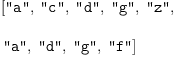(1)
Type: List(String)
fricas
b:= [1,7,8,9,2,4,6,17,18,14,32]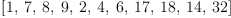(2)
Type: List(PositiveInteger?)
fricas
median a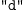(3)
Type: String
fricas
median b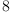(4)
Type: PositiveInteger?
fricas
mean b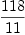(5)
Type: Fraction(Integer)
fricas
hmean b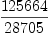(6)
Type: Fraction(Integer)
fricas
moment(b,1)(7)
Type: Fraction(Integer)
fricas
moment(b,2)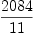(8)
Type: Fraction(Integer)
fricas
moment(b,3)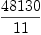(9)
Type: Fraction(Integer)
fricas
variance b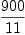(10)
Type: Fraction(Integer)
fricas
stdev b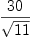(11)
Type: AlgebraicNumber?
fricas
gmean b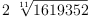(12)
Type: AlgebraicNumber?
fricas
center b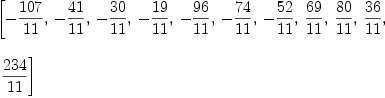(13)
Type: List(Fraction(Integer))
fricas
stdize b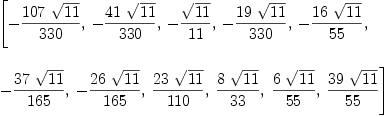(14)
Type: List(AlgebraicNumber?)
fricas
c := [3+7*%i,5+8*%i,8+3*%i,4+5*%i]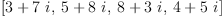(15)
Type: List(Complex(Integer))
fricas
mean c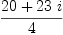(16)
Type: Fraction(Complex(Integer))

 Subject:   Be Bold !! ( 14 subscribers )